# How Do I Get the Number of Months Between Two Dates in Qlik?

• How-Tos FAQs
• October 21, 2020

## Number of Months Between Two Dates in Qlik

In Qlik Sense, there are no built-in functions that can show the number of months between two dates in Qlik, but you can solve this problem with the help of expression, calculate the dates in advance in the variables date_to and date_from:

``````=year('\$(date_to)')*12+month('\$(date_to)')-year('\$(date_from)')*12+month('\$(date_from)')

-

if(day('\$(date_from)')<day('\$(date_to)'),0,1)``````

The above calculation shows the number of months from the first Date subtracted from the number of months from the second Date, equalling the difference in months.

Add the condition in order to take into account the incomplete months.

## P() & E() and where do you use them?

P () and E () are used in set-analysis to filter one Field by values in another one, or even itself.

P or possible – considers the values in another field.

E or excluded – excludes the values in another field.

Example:

Sometimes, a situation arises when comparing indicators for two different periods (analysis period and comparison period). In this case, you can determine the key figure for the analysis period by selecting the calendar filter. And to calculate the indicator of the comparison period, you need to add a set analysis. Use the following expression for this case:

``{<Date = {"> = min (DateP) <= max (DateP)"}>}.``

However, this restriction does not always give the correct result (in this case, the entire interval is formed, but the goal may not always be the same). Therefore, a correct solution would be the following:

``{<Date = p (DateP)>}.``

The specified expression indicates that the indicator is calculated only for dates included in the DateP field.

When it is necessary, on the contrary, to use those values that are not related to the sample, it is necessary to use E (). When calculating the indicator and using

``{<Product = e (Product_e)>}.``

Here, the element function E () returns a list of values that are in the «Product» field but not in the «Product_e» field.

You can also use the P () function to exclude blank values from a field, for example, by writing.

``sum ({<item_name = p (item_ name)>} sales)``

We will calculate the turnover only for those products that have a name in the assortment reference book.

how to write !=(not equal to)

In set analysis, the combination – = is used to obtain the “not equal” logic.

In expressions, you must use the relational operator <>.

A numeric comparison is performed if both operands can be interpreted numerically. The operation returns a boolean value for evaluating the comparison result.

Converting date format

By default, the date format is set in the script on the main page. For example:

``SET DateFormat = 'DD.MM.YYYY';``

The Date () function allows you to convert an expression to a date using the specified format.

The syntax for the Date () function is:

Date (number [, format])

• number is the number to be changed
• format is the date format.

Examples of date formats: ‘YY-MM-DD’, ‘M / D / YY’, ‘DD.MM.YYYY’ etc.

Format number within expression

To change the number format, use the num () function

The syntax for the num () function is:

Num (number [, format [, dec_sep [, thou_sep]]]), where number is the number to be changed, format is the format to convert the number to, dec_sep is the decimal separator, thou_sep is the thousands separator.

You can specify decimal separator and thousands separator in the “Main” section of the load editor.

SET ThousandSep = ‘,’;

SET DecimalSep = ‘.’;

Examples of number formats: ‘#,##0’, ‘# ###,00’, ‘#,##0%’.

If you are not sure about which format mask to use and how it writes, look here:Using Greater Than in Set Analysis

In a set analysis, a condition is always defined through “=”; therefore, to pass a “greater than or equal” condition, it is necessary to write as follows:

``{<Date = {"> = min (DateP)"} >}``

Or, for example:

``{<Sales = {"> = 1000"} >}``

If you want to indicate dates interval in the set-analysis, we write like this:

``Date = {"> = min (DateP) <= max (DateP)"}``

QVD, QVW, and QVX

QVD – Qlikview Data

QVD is a file that contains an exported datasheet from Qlik products. QVD is a proprietary Qlik format, which, accordingly, can only be created and read in QlikView / Sense or some specialized third-party application, for example, EasyQlik QViewer. The file format is optimized for speed (10-100 times faster than from other sources) when reading data within a QlikView / Sense script, and at the same time, does not take up much space.

Files in this format consist of 2 parts:

• XML part: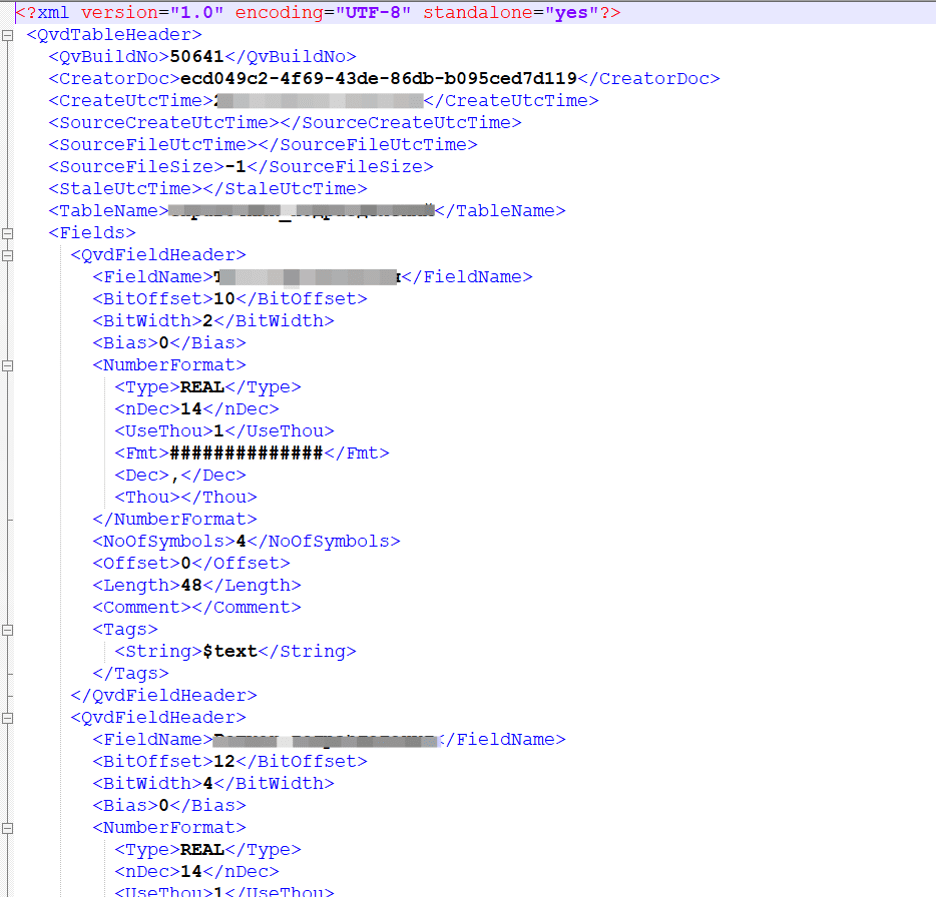• Data-part where data is stored in hashed form.

QVW – in Qlikview, or QVF – in Qlik Sense

These are QlikView / Sense app files that contain reports, charts, KPIs, load scenarios, expressions, and business settings used in data presentation.

QVX – Qlikview Data Exchange

QVX: Stands for Qlikview Data Exchange. You can make your connector to Qlikview files, mainly used if you’re not using ODBC/OLEDB.

Month number to Month name

If you need to convert the number (01, 02, 03, 04 …) of the month to text, you can use the expression:

date (date_field, ‘MMMMM’) – for long month name

Or

date (date_field, ‘MMM’) – for month abbreviation

where “date_field” – is a field, in data format. How who will indicate the name of the month is configured on the Main tab through the variables LongMonthNames and MonthNames, respectively.

Format Number (in Thousand)

Unfortunately, no built-in function allows you to convert a number to thousands (format “number K”).

In the Qlik script, you can set the NumericalAbbreviation variable, for example like this:

``SET NumericalAbbreviation='3:k;6:M;9:G;12:T;15:P;18:E;21:Z;24:Y;-3:m;-6:μ;-9:n;-12:p;-15:f;-18:a;-21:z;-24:y';``

However, this is only used on a specific list of visuals, such as a pie chart: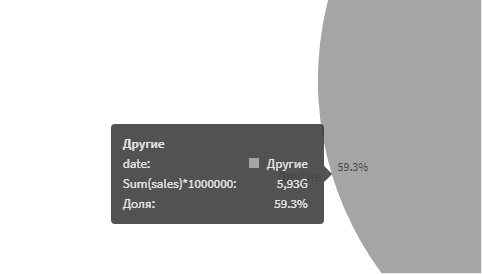But in the Table, the same expression will look different: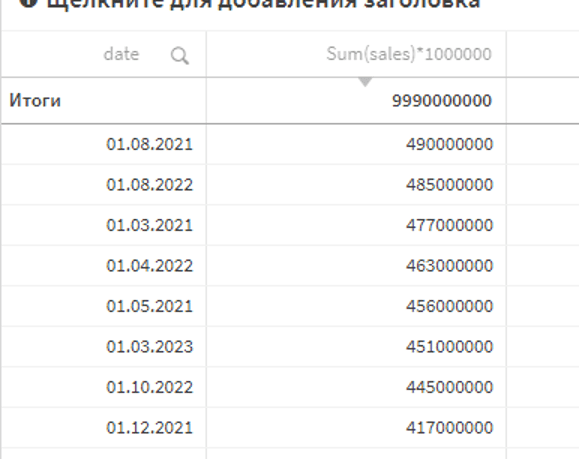If you need to make the necessary transformation work in the Table, you can make a calculation that will allow you to bring the number to the required form, for thousands, for example, it will be such an expression:

``num (Sum (sales) * 1000000/1000, '# ###. 00K')``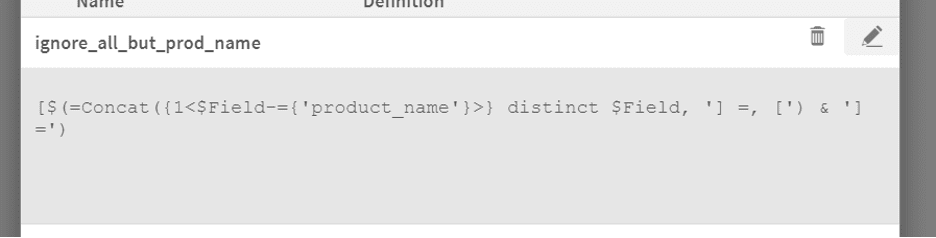Set analysis – ignores filters on all fields but one

It is often necessary to ignore selections in one Field, a set of fields, or all fields from one Table.

To do this, you can use Qlik’s ability to display system fields – \$ Field and tables – \$ Table.

The function for the set analysis will look like this:

To ignore specific field/fields:

``[\$ (= Concat ({1 <\$ Field = {'field_to_ignore1', 'field_to_ignore2',…, 'field_to_ignoreN'}>} distinct \$ Field, '] =, [') & '] =')``

To ignore all fields except a specific one:

``[\$ (= Concat ({1 <\$ Field - = {'field_to_keep1', 'field_to_keep2',…, 'field_to_keepN'}>} distinct \$ Field, '] =, [') & '] =')``

To ignore specific table/tables:

``[\$ (= Concat ({1 <\$ Table = {'table_to_ignore1', 'table_to_ignore2',…, 'table_to_ignoreN'}>} distinct \$ Field, '] =, [') & '] =')``

To ignore all fields except a specific one:

``[\$ (= Concat ({1 <\$ Table - = {'table_to_keep1', 'table_to_keep2',…, 'table_to_keepN'}>} distinct \$ Field, '] =, [') & '] =')``

You can put those expressions in variable or straight in set-analysis expression.

For example, we have such  data model: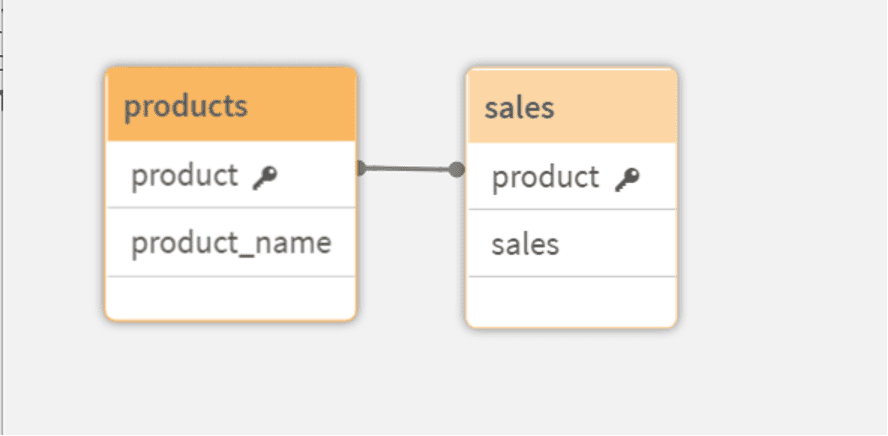If we want to have the sum of sales, where we will ignore filters for all fields, but “product_name,” we can create a variable:And then put it in set-analysis expression: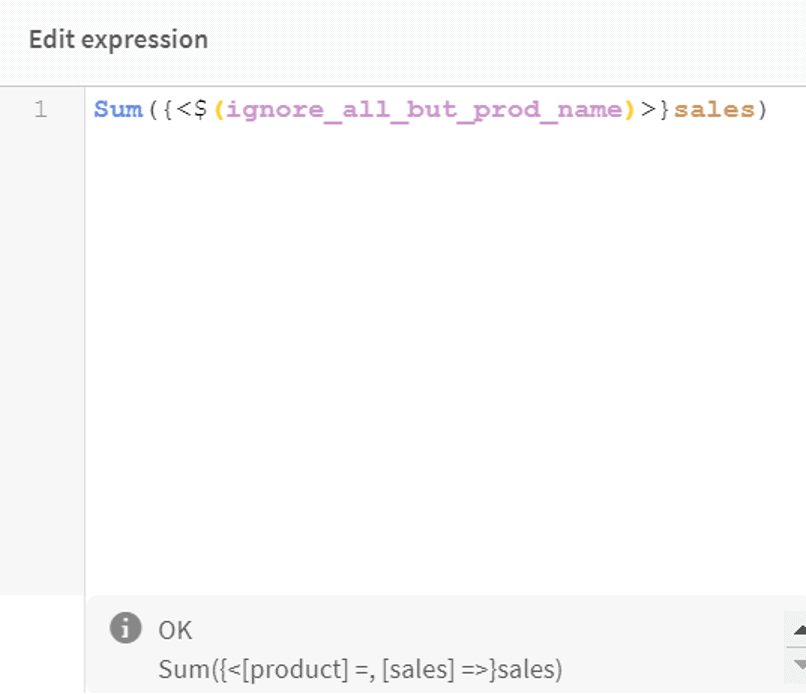``Sum({<\$(ignore_all_but_prod_name)>}sales)``

As you can see; as a result, we will have all our fields filters ignored, but the one we wanted to keep. That is how we can find the number of months between two dates in Qlik?

### No-Code Data Transformation

Model data directly in your Snowflake instance with easy-to-use functions and visual workflows

## More Resources We Think You Might Like

### How Do I Get the Number of Months Between Two D...

Number of Months Between Two Dates in Qlik In Qlik Sense, there are no built-in functions that ca...

• How-Tos FAQs
• October 21, 2020

### How to Start Loving the Qlik Synthetic Key

Have you learned to love the Qlik Synthetic key without worry? Love Qlik synthetic key? What a th...

• How-Tos FAQs
• October 27, 2020

### What else can the Qlik Peek function do?

Qlik Peek function – what else can it do? We most commonly use the peek() function in Qlik, to cr...

• How-Tos FAQs
• October 26, 2020
[class^="wpforms-"]
[class^="wpforms-"]
[class^="wpforms-"]
[class^="wpforms-"]
[class^="wpforms-"]
[class^="wpforms-"]
[class^="wpforms-"]
[class^="wpforms-"]
[class^="wpforms-"]
[class^="wpforms-"]